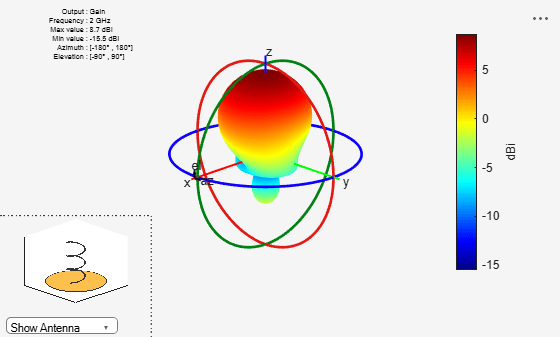## Far-field Terminologies### Directivity

Directivity is the ability of an antenna or array to radiate power in a particular direction. It can be defined as ratio of maximum radiation intensity in the desired direction to the average radiation intensity over all the directions. The equation for directivity is:

`$D=\frac{4\pi U\left(\theta ,\varphi \right)}{{P}_{rad}}$`

where:

• D is the directivity of the antenna.

• U is the radiation intensity of the antenna as a function of direction.

• Prad is the average radiated power of the antenna over all the directions.

Antenna directivity is dimensionless and is calculated in decibels compared to the isotropic radiator (dBi).

#### Directivity Value of Helix Antenna.

Calculate the directivity of a helix antenna.

```h = helix; D = pattern(h, 2e9, 0, 1:1:360);```

Showing the first five directivity values.

`Dnew = D(1:5)`
```Dnew = 5×1 -6.4066 -6.1929 -5.9655 -5.7262 -5.4770 ```

### Gain

The gain of an antenna or array depends on the directivity and efficiency of the antenna or array. It can be defined as the ratio of maximum radiation intensity in the desired direction to the total power input of the antenna. The equation for gain of an antenna is:

`$G=\frac{4\pi U\left(\theta ,\varphi \right)}{{P}_{in}}$`

where:

• G is the gain of the antenna.

• U is the radiation intensity of the antenna.

• Pin is the total power input to the antenna.

If the efficiency of the antenna in the desired direction is `100%`, then the total power input to the antenna is equal to the total power radiated by the antenna, that is, ${P}_{in}={P}_{rad}$. In this case, the antenna directivity is equal to the antenna gain.

#### Gain of Helix Antenna

Calculate the gain of the helix antenna.

```h = helix; pattern(h, 2e9, 'Type',"gain")```### Realized Gain

The realized gain or absolute gain of an antenna or array takes into account reflection (mismatch) losses. The port mismatch losses arise due to the difference in antenna input impedance and matching impedance. In figure above,

• Pin is the input power to the antenna.

• Prad is the radiated power of the antenna.

• Pref is the reflected power from antenna due to the port mismatch.

This figure shows relation between gain, realized gain, and directivity as follows:

Realized Gain = (Port efficiency) * (Radiation efficiency) * (Gain) = (Port efficiency) * (Directivity)

Port efficiency = Pref / Pin

#### Realized Gain of Helix Antenna

Calculate the realized gain of the helix antenna.

```h = helix; pattern(h,2e9,'Type',"realizedgain");```Makarov, Sergey N. Antenna and EM Modeling in MATLAB. Chapter3, Sec 3.4 3.8. Wiley Inter-Science.

 Balanis, C.A. Antenna Theory, Analysis and Design, Chapter 2, sec 2.3-2.6, Wiley.